Home | | Engineering Physics II | Semiconducting Materials

# Semiconducting Materials

1 Introduction 1.1. Properties of Semiconducting materials 1.2. Elemental and Compound semiconductors 2 Intrinsic Semiconductors – Electrons and Holes 3 Carrier Concentration in an Intrinsic Semiconductor 3.1. Density of Electrons 3.2. Density of Holes 3.3. Density of intrinsic Carrier Concentration 3.4. Fermi Energy Level 3.5. Electrical Conductivity 3.6. Determination of Band gap in intrinsic semiconductor 4 Extrinsic Semiconductor 4.1. n- type Semiconductor 4.2. p- type Semiconductor 5 Carrier Concentration and Fermi level Calculation in n- type Semiconductor 5.1. Fermi energy level 5.2. Density of electron Concentration 5.3. Electrical Conductivity 5.4. Variation of carrier concentration with temperature in n – type semiconductor 6 Carrier Concentration and Fermi level Calculation in p- type Semiconductor 6.1. Carrier Concentration 6.2. Fermi Energy level 7 Variation of carrier concentration with temperature in P- type Semiconductor 8 Hall Effect 8.1. Statement 8.2. Theory 8.3. Calculation of Hall Coefficient 8.4. Applications of Hall Effect

SEMICONDUCTING MATERIALS

1 Introduction

1.1. Properties of Semiconducting materials

1.2. Elemental and Compound semiconductors

2 Intrinsic Semiconductors – Electrons and Holes

3 Carrier Concentration in an Intrinsic Semiconductor

3.1. Density of Electrons

3.2. Density of Holes

3.3. Density of intrinsic Carrier Concentration

3.4. Fermi Energy Level

3.5. Electrical Conductivity

3.6. Determination of Band gap in intrinsic semiconductor

4 Extrinsic Semiconductor

4.1. n- type Semiconductor

4.2. p- type Semiconductor

5 Carrier Concentration and Fermi level Calculation in n- type Semiconductor

5.1. Fermi energy level

5.2. Density of electron Concentration

5.3. Electrical Conductivity

5.4. Variation of carrier concentration with temperature in n – type semiconductor

6 Carrier Concentration and Fermi level Calculation in p- type Semiconductor

6.1. Carrier Concentration

6.2. Fermi Energy level

7 Variation of carrier concentration with temperature in P- type Semiconductor

8 Hall Effect

8.1. Statement

8.2. Theory

8.3. Calculation of Hall Coefficient

8.4. Applications of Hall Effect

1 INTRODUCTION

Based on electrical resistance

The resistivity of a semiconductor is lesser then an insulator but more than that of a conductor. It is in the order of 10-4 to 0.5 ohm metre.

Based on energy band

A semiconductor has nearly empty conduction band and almost filled valance band with very small energy gap (≈ 1eV).1.1. GENERAL PROPERTIES OF SEMICONDUCTORS

They are formed by covalent bonds.

They have an empty conduction band at 0K They have almost filled valance band

They have small energy gap They posses crystalline structure

They have negative temperature co efficient of resistance

If the impurities are added to a semiconductor, its electrical conductivity increases. Similarly, if the temperature of the semiconductor increased, its electrical conductivity increases.

1.2. ELEMENTAL AND COMPOUND SEMICONDUCTORS

The semiconductors are classified mainly into two types based on composition of materials.

Elemental semiconductors

Compound semiconductors

Elemental semiconductors

These semiconductors are made from a single element of fourth group elements of the periodic table.

They are also known as indirect band gap semiconductors

Example –Germanium, silicon

Compound semiconductors

Semiconductors which are formed by combining third and fifth group elements or second and sixth group elements in the periodic table are known as compound semiconductors. These compound semiconductors are also known as direct band gap semiconductors.

Example –1. Combination of third and fifth group elements

Gallium phosphide (GaP)

Gallium arsenide (GaAs)

Indium phosphide (InP)

Indium arsenide (InAs)

2. Combination of second and sixth group elements Magnesium oxide (MgO)

Magnesium silicon (MgSi) Zinc oxide (ZnO)

Zinc sulphide (ZnS)

Types of semiconductors

Based on the purity semiconductors are classified in to the following two types. Intrinsic semiconductors

Extrinsic semiconductors

Intrinsic semiconductors

A semiconductor in extremely pure form, without the addition of impurities is known as intrinsic semiconductors. Its electrical conductivity can be changed due to thermal excitation.

At 0K the valance band is completely filled and the conduction band is empty. The carrier concentration (i.e) electron density (or) hole density increases exponentially with increase in temperature.

Extrinsic semiconductors

A semiconductor in extremely impure form, with the addition of impurities is known as extrinsic semiconductors.

2 INTRINSIC SEMICONDUCTORS –ELECTRONS AND HOLES

We know that, at 0K intrinsic pure semiconductor behaves as insulator. But as temperature increases some electron move from valance band to conduction band as shown in fig. therefore both electrons in conduction band and holes in valance band will contribute to electrical conductivity. Therefore the carrier concentration (or) density of electrons (ne) and holes (nh) has to be calculated.Assume that electron in the conduction band as a free electron of mass m*e and the hole in the valance band behave as a free particle of mass m*h. The electrons in the conduction band have energies Lying from   Ec   to   ∞   and   holes-∞intotheEv  valanceasshownb Ec represents the lowest energy level of conduction band Ev represents the highest energy level of the valance band.

3. CARRIER CONCENTRATION IN AN INTRINSIC SEMICONDUCTOR

3.1. DENSITY OF ELECTRONS IN CONDUCTION BANDWe know Fermi function, probability of finding an electron in a given energy state isSubstituting equation 4 & 3 in equation 1 we have Density of electrons in conduction band within the limits Ec to ∞ asSince to move an electron from valavce band to conduction band the energy required is greater then 4 KBT  (i.e) E –EF >>KBT (or) (E - EF) / KBT >>1Let us assume that E –Ec = x KBT

Or E = Ec + x KBT

Differentiating we get dE = KBT. dx,

Limits: when E = Ec; x = 0

When   E   =   ∞;   x   =   ∞

Limits are 0 to ∞

Equation 6 can be written as3.2. DENSITY OF HOLES IN VALANCE BAND

We know F(E) represents the probability of filled states. As the maximum probability 1, the probability of un filled states will be [1 –F(E)].

Example, if F(E) = 0.8 then 1 –F(E) = 0.2

(i.e) 80% chance of finding an electron in valance band and 20% chance of finding a hole in valance band.

Let the maximum energy in valance band be Ev and the minimum energy be -∞. Theref density of hole in valance band nh is given byLet us assume that

Ev –E = x KBT,

Differentiating we get  dE = - KBT. dx,

Limits: when E =   ∞;

When Ev –(-∞)   =   x;   x   =   ∞

when E = Ev; x = 0

Limits   are   ∞   to   0

Equation 11 becomes3.3. DENSITY OF INTRINSIC CARRIER CONCENTRATION

In an intrinsic semiconductor, the concentration of electrons in the conduction band is equal to the concentration of holes in the valance band.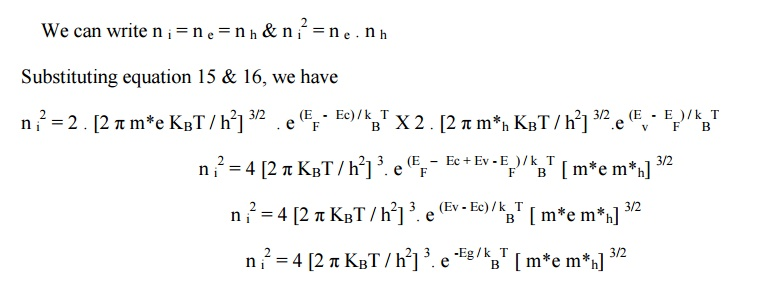Where Ec –Ev = Eg is the forbidden energy gap.3.4. FERMI ENERGY LEVEL

For an intrinsic semiconductor number of electrons (i.e) electron density will be the same as that of the number of holes (i.e) hole density.

(i.e)  n e = n h

Equating equations 7& 12If m*h = m*e, then log m*h / m*e = 0 since log 1 = 0

(i.e) the Fermi energy level lies in the midway between Ec and Ev as shown fig (since at 0K, T = 0).But in actual case m*h m*e and the Fermi energy level slightly increases with increase in temperature as shown in fig.Mobility

When an electric field is applied to a semiconductor, the charge carrier namely electrons and holes attain drift velocity Vd. The drift velocity attained by the charge carrier is proportional to electric field strength E.

Drift velocity Vd is proportional to E

(i.e)  Vd α     E

Vd =   μ   E

Where μ is proportionality constant and it is known as the mobility of the charge carrier being  considered. Mobility μ is defined as the velocity of a charge carrier produced unit field strength.The electron and hole nobilities are denoted by the symbols μe and μh  .

3.5.ELECTRICAL CONDUCTIVITY

Electrical conductivity of a semiconductor is different from that of a conductor. The charge carriers in a conductor are electrons. In the case of a semiconductor, both electron and holes are charge carriers.

On the basis of free electron theory of solids, the electrical conductivity of metal is given by

σ =μ  ne        ----(1)

Using the equation 1, the electrical conductivity of a semiconductor due to electrons in the conduction band is given by

σe  = n e e μ e             -----(2)

Where n e is the number of electrons per unit volume; e is the charge of an electron

μe is the electron mobility.

Similarly, the electrical conductivity of a semiconductor due to holes in the valance band is given by

σh = n h e μ h              ----(3)

where n h - is the hole density in the valance band; e - is the charge of a hole

μh –is the hole mobilityTherefore the total electrical conductivit conductivities due to electrons and holes.

σi =  σ e +  σ h          ---------(4)

Substituting equations 2& 3 in the equation 4,

We haveFor an intrinsic semiconductor, the number of electrons in the conduction band is equal to the number of holes in the valance band,

(i.e) n e = n h = n i

Where n i is the intrinsic carrier concentration.

Hence, the electrical conductivity of an intrinsic semiconductor is given by

σi = e[ n i μe + n i μh]

Thus, the electrical conductivity depends upon the negative exponential of the forbidden energy gap  Eg and on the motilities’ of both electron and holes.

3.6. BAND GAP DETERMINATION

Electrical conductivity of an intrinsic semiconductor is given byThis equation (6) is similar to the equation of a straight line, y = mx + c

In equation (6), y = log R; x = 1 / T;  m = Eg / 2 KBT and c = logC

From  the  equation  6,  it  is  noted  that  by  measuring  the  resistance  of  an  intrinsic semiconductor at different temperatures, its forbidden energy gap Eg can be determined.

If a graph is drawn between log R and 1 / T, the value of Eg is determined from the slope of a straight line.Slope = Eg / 2k

Eg = 2k X slope          ----(7)

From the graph slope = dy / dx

Substituting the expression for the slope in equation (7), we have

Eg = 2k dy / dx

Where, k is the Boltzmann’s   constant.

4 EXTRINSIC SEMICONDUCTOR

In a semiconducting material, if the charge carriers originate from impurity atoms added to the original material, then this type of semiconductor is called extrinsic or impure semiconductor. It is also known as doped semiconductor.

Doping

The method of adding impurities to a pure semiconductor is known as doping and impurity added is called doping agent or dopant.

The addition of impurities will increase the number of free electrons and holes in a semiconductor and hence increases its electrical conductivity.

Conductivity is high.

Conductivity can be controlled to any desired value through the control of doping concentration.

Conductivity is not a function of temperature.

Types of extrinsic semiconductor

The extrinsic semiconductors are classified into two types based on the type of impurity added.

(i). n –type semiconductor

(ii). P –type semiconductor

4.1. N –TYPE SEMICONDUCTOR

When a small amount of pentavalent impurity (group V element) is added to a pure semiconductor, it becomes a n –semiconductor.. such impurities are known as donor impurities because they donate the free electrons to the semiconductor crystal.

A  pentavalent  impurity  (arsenic)  having  five  valance  electrons  is  added  to  a  pure

semiconducting material having four valance electrons (silicon or germanium).

The four valance electrons of the impurity atoms bond with four valance electrons of the

semiconductor atom and remaining 1 electron of the impurity atom is left free as shown fig.

Therefore number of free electrons increases, as the electrons are produced in excess, they the majority charge carrier in n –type semiconductor and holes are the majority charge carriers.

Since electrons are donated in this type of semiconductor the energy level of these donated electrons is called donor energy level (Ed) as shown in fig.

Ed is very close to conduction band and hence even at room temperature the electrons are easily excited to conduction band. The current flow in this type of semiconductor is due to electrons.4.2. P –TYPE SEMICONDUCTOR

P –type semiconductor is obtained by doping an intrinsic semiconductor with trivalannt (3 electrons in valance band)impurity atoms like boron, gallium, indium etc.,

The three valance electrons of the impurity atom pairs with three valence electrons of the semiconductor atom and one position of the impurity atom remains vacant, this is called hole as shown in fig

Therefore the number of holes increased with impurity atoms added to it. Since holes are produced in excess, they are the majority charge carriers in p –type semiconductor and electrons are the minority charge carriers.

Since the impurity can accept the electrons this energy level is called acceptor energy level (Ea) and is present just above the valence band as shown in fig.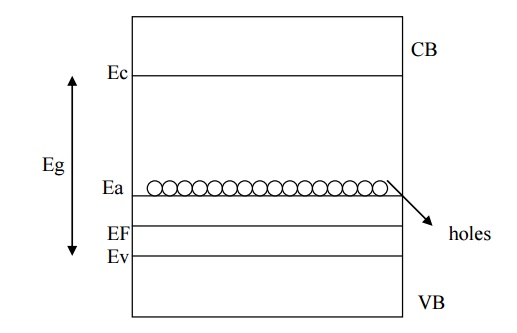Here the current conduction is mainly due to holes (holes are shifted from one covalent bond to anther).

5 CARRIER CONCENTRATION IN N –TYPE SEMICONDUCTOR

5.1. FERMI ENERGY LEVEL

The energy band structure of n –type semiconductor is shown in fig. At 0 K, EF will lie exactly between Ec and Ed, but even at low temperature some electrons may go from Ed to Ec. Let us assume that Ec –EF > KBT. Then the density of electrons in conduction band can be written as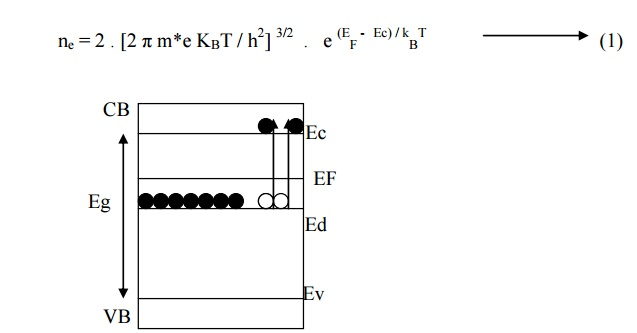Let Nd be the number of donor energy levels per cm 3 (i.e) density of state Z(Ed) dE. If some electrons donated from donor energy level to conduction band say for example if two electrons goes to conduction band then two vacant sites (holes) will be created in Ed levels.

Thus in general we can write the density of holes in donor energy level as

N(Ed) dE = Z(Ed) dE .(1 –F(Ed))

(i.e) n h = Nd .(1 –F(Ed))               ---(2)At equilibrium condition

Number of electrons per unit volume in conduction band (electron density) = Number of holes per unit volume in conduction band (hole density)

Equating equation (1) and equation (5) we get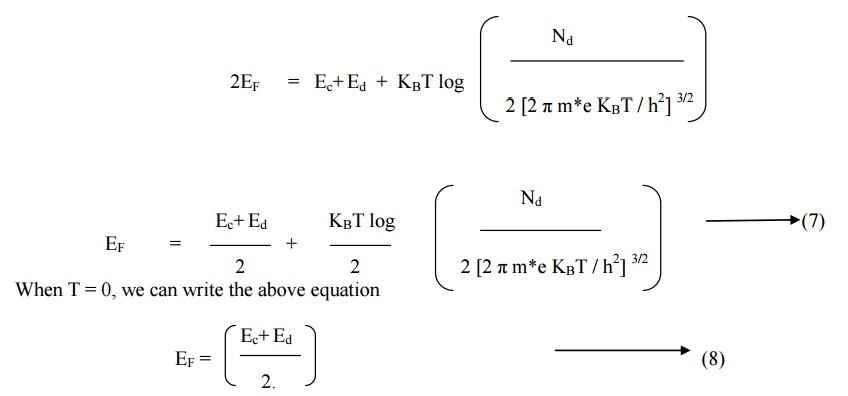Equation (7) shows that, at 0K, EF will lie expertly in the midway between Ec and Ed.

5.2 DENSITY OF ELECTRON CONCENTRATION (n)

By substituting Ef value from equation (7) into expression5.3ELECTRICAL CONDUCTIVITY

We know that electrical conductivity in semiconductor is given by

σn = n e e μ e +n h e μ h

where μh=0

σn = n e e μ e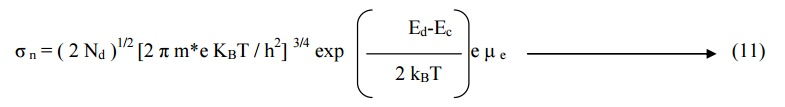This equation represent the electrical conductivity of n –type semiconductor.

5.4 VARIATION OF    CARRIER CONCENTRATION WITH TEMPERATURE  IN N – TYPE SEMICONDUCTOR

When the temperature increased some electrons in Ed level may be shifted to conduction band and hence some vacant sites will be created in Ed levels. Therefore the Fermi level shifts down to separate that empty levels and the filled valance band level as shown in fig.From the above figure it can be seen that for the same temperature, if the impurity atoms (i.e) doping level is increased, the electron concentration increases and hence Fermi level increases.

6 CARRIER CONCENTRATION AND FERMI LEVEL IN P –TYPE SEMICONDUCTOR

6.1. CARRIER CONCENTRATION

For p –type at absolute zero EF will be exactly between Ea and Ev. At low temperature some electron from valence band fills the holes in the acceptor energy level as shown in figure.

We know the density of holes in the valence band,Let Na be the number of acceptor energy levels per cm 3 which has energy Ea above valence band. If some electrons are accepted by acceptor energy levels from the valence band, say for example

if two electrons are accepted to fill the hole sites in the acceptor levels, then two holes will be created in the valence band as shown in fig .Therefore in general the electron density in the acceptor energy level can be written as

N(Ea) dE = Z(Ea) dE  F(Ea)At equilibrium condition

Number of electrons per unit volume in conduction band (electron density) = Number of holes per unit volume in conduction band (hole density)

Equating equation (1) and equation (4) we get6.2. FERMI ENERGY LEVEL

At 0K, when T= 0 we can write equation (5)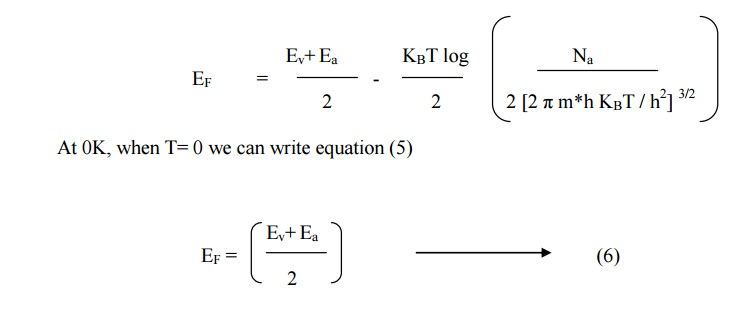7 VARIATION OF FERMI LEVEL WITH TEMPERATURE AND IMPURITY CONCENTRATION IN P –TYPE SEMICONDUCTOR

When the temperature is increased, some of the electrons in the valence band will go to the acceptor energy level by breaking up the covalent bonds and hence Fermi energy level shifted upward direction for doping level of Nd = 10 21 atoms / m3 as shown in figure.

From the figure it can be seen that for the same temperature, if the impurity atoms (i.e) doping level is increased say Nd = 10 24 atoms /m3, the hole concentration increases and hence the Fermi level decreases.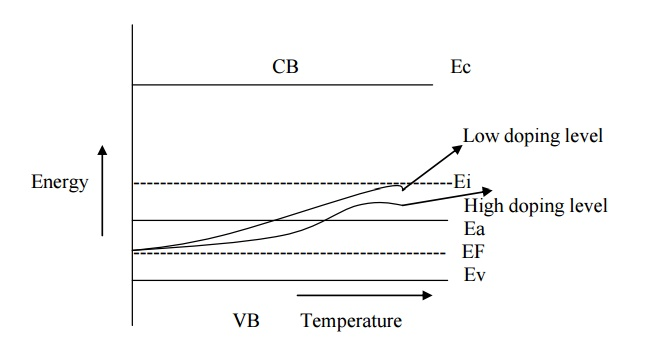Therefore at low temperature the Fermi energy level may be increased up to the level of intrinsic energy level (Ei).

8 HALL EFFECT:

Measurement of conductivity will not determine whether the conduction is due to electron or holes and therefore it will be very difficult to distinguish between p –type and n- type semiconductors.

Therefore Hall Effect is used to distinguish between the two types of charge carriers and their carrier densities and is used to determine the mobility of charge carriers.

8.1.STATEMENT

When a conductor (metal or semiconductor) carrying current (I) is placed perpendicular to a magnetic field (B), a potential difference (electric field) is developed inside the conductor in a direction perpendicular to both current and magnetic field. This phenomenon is known as Hall Effect and the voltage thus generated is called Hall voltage.

8.2. THEORY

Hall effect in n- type semiconductor

Let us consider a n-type semiconductor material in the form of rectangular slab. In such a material current flows in X –direction and magnetic field B applied in Z- direction. As a result, Hall voltage is developed along Y –direction as shown in figure.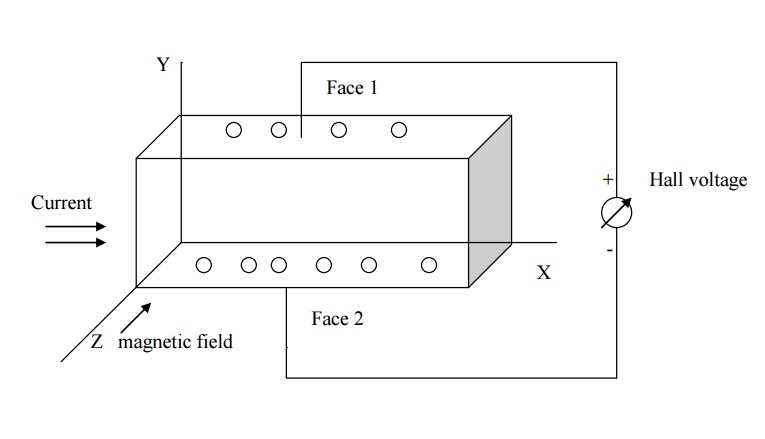Since the direction of current is from left to right the electrons moves from right to left in X-direction as shown in fig

Now due to magnetic field applied the electron moves towards downward direction with velocity v and cause negative charge to accumulate at face (1) of the material as shown in fig. therefore the potential difference is established between the face (2) and face (1) of the specimen which gives rise to EH in the negative Y direction.Here, the force due to potential difference = -e EH          ---(1)

Force due magnetic field = - Bev         ---(2)

At equilibrium equation (1) = equation (2)

-e EH = - Bev

EH = Bv                       ---(3)

We know the current density Jx in the X- direction is

Jx = -ne ev

v = - Jx / ne e                   ---(4)

Substituting equation (4) in equation (3) we get

EH = - B Jx / ne e                      ----(5)

EH = RH . Jx . B     ----(6)

Where RH is known as the Hall co –efficient, is given by

RH = - (1 / ne e)              ----(7)

The negative sign indicates that the field is developed in the negative Y –direction.

Hall effect in p –type semiconductor

Let us consider a p –type semiconducting material for which the current is passed along X – direction from left to right and magnetic field is applied along Z –direction as shown in fig. since the direction of current is from left to right, the holes will also move in the same direction as shown in fig.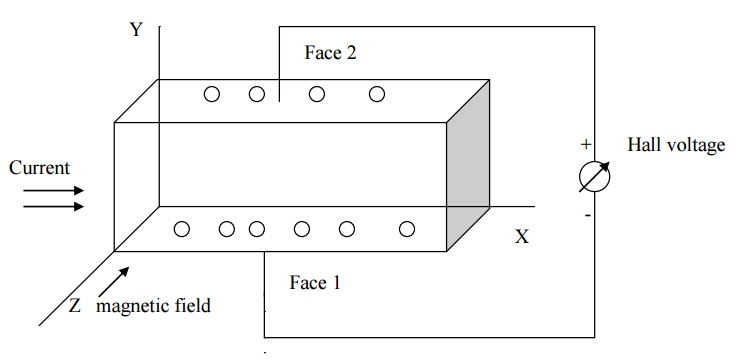Now due to magnetic field applied the holes moves towards downward direction with velocity v and accumulates at the face (1). A potential difference is established between face (1) and (2) in the positive Y - direction.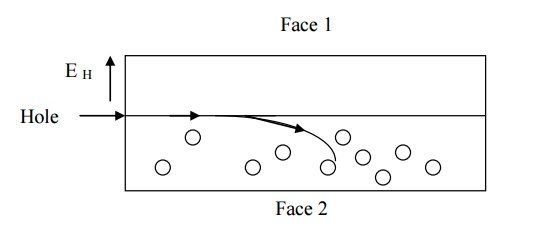Here, the force due to potential difference = -e E       ----(8)

Force due magnetic field = Bev                  ----(9)

At equilibrium equation (1) = equation (2)

e EH =  Bev

EH = Bv        --------------(10)

We know the current density Jx in the X- direction is

Jx = nh ev

v = Jx / nh e               -----------(11)

Substituting equation (4) in equation (3) we get

EH = B Jx / nh e

EH = RH . Jx . B

Where RH is known as the Hall co –efficient, is given by

RH = (1 / nh e)          ----(12)

The positive sign indicates that the field is developed in the positive Y –direction

Hall coefficient in terms of hall voltage

If the thickness of the sample is t and the voltage developed is VH, then

Hall voltage VH = EH .t          ---((13))

Substituting equation (6 )in equation (13), we have

VH = RH Jx B .t                      ----(14)

If b is the width of the sample then

Area of the sample = b.t

Current density = Jx = Ix / bt                    -----(15)

Substituting equation (15) in equation (14) we get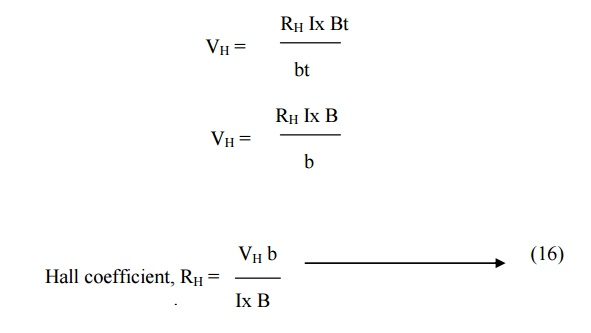The sign for VH will be opposite for n and p type semiconductors.

Mobility of charge carriers

We know that Hall coefficient,

RH = - (1 / ne e)

The above expression is valid only for conductors where the velocity is taken as the drift velocity.

But for semiconductor velocity is taken as average velocity so RH for n- type semiconductor is modified as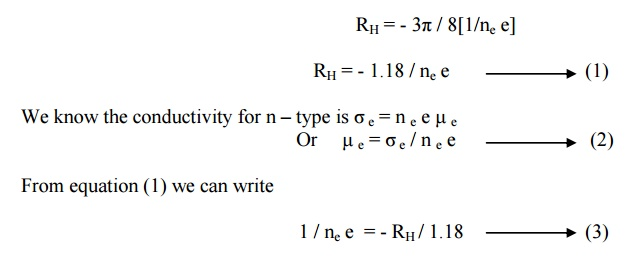Substituting equation (3) in equation (2) we getFor n –type semiconductor, the mobility of electron isSimilarly for p –type semiconductor, the mobility of hole is8.3. EXPERIMENTAL DETERMINATION OF HALL EFFECT

A semiconducting material is taken in the form of a rectangular slab of thickness t and breadth b. A suitable current Ix ampere is passed through this sample along X- axis by connecting it to a battery.This sample is placed in between two poles of an electro magnet such that magnetic field is applied along Z –axis.

Due to Hall Effect, Hall voltage (VH) is developed in the sample. This voltage measured by fixing two probes at the centers of the bottom and top faces faces of the sample. By measuring Hall voltage, Hall coefficient is determined from the formulaFrom the hall coefficient, carrier concentration and mobility can be determined.

8.4. APPLICATIONS OF HALL EFFECT

It is used to determine whether the material is p-type or n-type semiconductor. (ie ) if RH is negative then the material n-type. If the RH is positive then the material p-type.

It is used to find the carrier concentration

It is used to find the mobility of charge carriers μe, μh.

It is used to find the sign of the current carrying charges.

It is used to design magnetic flux meters and multipliers on the basis of Hall voltage. It is used to find the power flow in an electromagnetic wave.

Study Material, Lecturing Notes, Assignment, Reference, Wiki description explanation, brief detail
Physics : Semiconducting Materials : Semiconducting Materials |